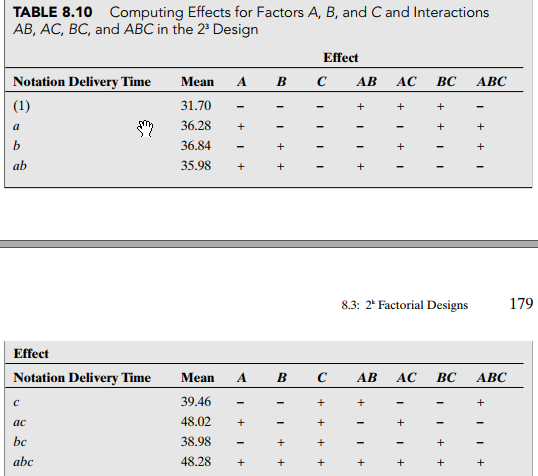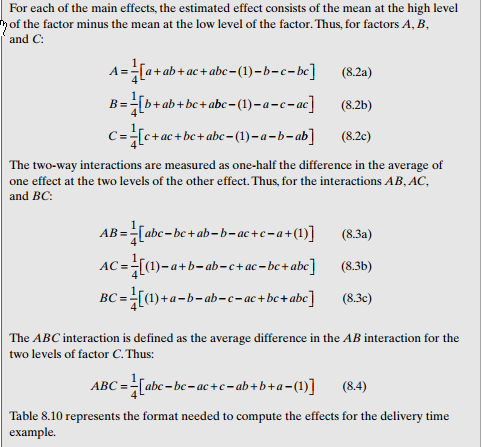## Simulate responses for fractional factorial design

Hello,

I am learning DoE  and want to simulate responses for fractional factorial design.

As an input for simulation I want to use estimated effect sizes of main factors and some interactions.

I know there is a formula for calculating effects from obtained responses:

"For each of the main effects, the estimated effect consists of the mean at the high level of the factor minus the mean at the low level of the factor."

Here is the screenshot of fractional factorial design from a book:And here is formulas for calculation of effects when responses are known:But I want inverse:

1. Input values of estimated main effects and interactions (A B C AB AC etc.)
2. Derive a simulated responses for all rows (1, a, b, ab..) so that they lead to that predefined effects.

Could you help me please how to approach this problem?

SAS, R or JMP code would be extremely helpful.

After all I want to use derived responses in this SAS JMP code for simulation binomial responses. Also I will use it with higher number of factors.

3 REPLIES 3

## Re: Simulate responses for fractional factorial design

To simulate data for such a situation, you have to first write out (or have in your mind) an actual model. For example, y = 2 + 5*A – 6*B + 4*A*C + error. Then the simulation ought to be simple (in SAS, you'd need to choose one of the many functions that generate random variables for the error)

--
Paige Miller

## Re: Simulate responses for fractional factorial design

Hi @PaigeMiller,

For full factorial design in case I take Y as a success rate my prediction equation is:

0.12125 + Match( :A, -1, -0.04875, 1, 0.04875, . )
+Match( :B, -1, -0.06375, 1, 0.06375, . )
+Match( :C, -1, -0.00375, 1, 0.00375, . )
+Match( :A,
-1, Match( :B, -1, 0.02625, 1, -0.02625, . ),
1, Match( :B, -1, -0.02625, 1, 0.02625, . ),
.
) + Match( :A,
-1, Match( :C, -1, 0.00625, 1, -0.00625, . ),
1, Match( :C, -1, -0.00625, 1, 0.00625, . ),
.
) + Match( :B,
-1, Match( :C, -1, 0.00625, 1, -0.00625, . ),
1, Match( :C, -1, -0.00625, 1, 0.00625, . ),
.
)

How do I simulate responses based on this model for fractional factorial res III design?

After all I would like to use this responses in this script suggested on JMP forum. Also the actual number of factors I will use is 18 and res iV. The example above is for simplicity.

```New Table( "Untitled",
New Column( "A", Numeric, "Nominal", Format( "Best", 12 ),
Formula( Random Integer( 0, 1 ) ) ),
New Column( "B", Numeric, "Nominal", Format( "Best", 12 ),
Formula( Random Integer( 0, 1 ) ) ),
New Column( "C", Numeric, "Nominal", Format( "Best", 12 ),
Formula( Random Integer( 0, 1 ) ) ),
New Column( "Success?", Numeric, "Nominal", Format( "Best", 12 ),
Formula(
If(
:A == 0 & :B == 0 & :C == 0, Random Binomial( 1, 0.05 ),
:A == 0 & :B == 0 & :C == 1, Random Binomial( 1, 0.05 ),
:A == 0 & :B == 1 & :C == 0, Random Binomial( 1, 0.065 ),
:A == 0 & :B == 1 & :C == 1, Random Binomial( 1, 0.065 ),
:A == 1 & :B == 0 & :C == 0, Random Binomial( 1, 0.055 ),
:A == 1 & :B == 0 & :C == 1, Random Binomial( 1, 0.055 ),
:A == 1 & :B == 1 & :C == 0, Random Binomial( 1, 0.075 ),
:A == 1 & :B == 1 & :C == 1, Random Binomial( 1, 0.075 ),
Random Binomial( 1, 0.05 ) ) ) ) )```

## Re: Simulate responses for fractional factorial design

It looks to me like you are using JMP (or some other language) and not SAS, in which case I can't help you. If you are using JMP, they have their own community for asking questions.

it also appears to me that you have changed from talking about arbitrary Y variable to one that has to be a value between 0 and 1, is that correct?

--
Paige Miller
Discussion stats
• 3 replies
• 950 views
• 2 likes
• 2 in conversation